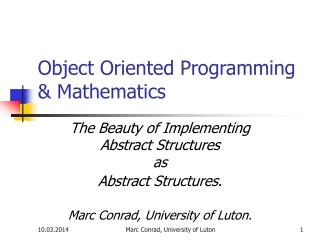DownloadDownload PresentationObject Oriented Programming & Mathematics

# Object Oriented Programming & Mathematics

Télécharger la présentation## Object Oriented Programming & Mathematics

- - - - - - - - - - - - - - - - - - - - - - - - - - - E N D - - - - - - - - - - - - - - - - - - - - - - - - - - -
##### Presentation Transcript

1. Object Oriented Programming & Mathematics The Beauty of Implementing Abstract Structures as Abstract Structures. Marc Conrad, University of Luton. Marc Conrad, University of Luton

2. Once upon a time... Mathematics before the 20th century Marc Conrad, University of Luton

3. there was two branches of Mathematics axiomatic Mathematics before the 20th century algorithmic Marc Conrad, University of Luton

4. Axiomatic Hilbert, N. Bourbaki, etc. axiomatic Mathematics before the 20th century algorithmic Marc Conrad, University of Luton

5. Algorithmic axiomatic Mathematics before the 20th century algorithmic Turing, Church, etc. Marc Conrad, University of Luton

6. Pure Mathematics & Computer Science axiomatic "pure" mathematics Mathematics before the 20th century algorithmic computer science Marc Conrad, University of Luton

7. With links in between. axiomatic "pure" mathematics Mathematics before the 20th century computer algebra etc. algorithmic computer science Marc Conrad, University of Luton

8. However, some topics of Computer Science seemed to be unrelated to mathematics... axiomatic "pure" mathematics Mathematics before the 20th century algorithmic computer science software design operating systems Marc Conrad, University of Luton

9. as e.g. object oriented programming. axiomatic "pure" mathematics Mathematics before the 20th century algorithmic object oriented programming is a technique to solve the "software crisis". It evolved in a context completely unrelated to mathematics. computer science e.g. object oriented programming Marc Conrad, University of Luton

10. But object oriented programming is closer to "axiomatic" mathematics than it appeared in the first place. object oriented programming... ... allows to implement abstract structures in an "axiomatic" way. "pure" mathematics axiomatic Mathematics before the 20th century algorithmic object oriented programming computer science Marc Conrad, University of Luton

11. We cannot implement: addition negation multiplication inversion "zero" "one" check if zero We can implement: subtraction (because of addition and negation) exponentiation a embedding of Z, Q. check for equality polynomials over this ring etc. Example: A ring (abstract). n Marc Conrad, University of Luton

12. We cannot implement: addition negation multiplication inversion "zero" "one" check if zero We can implement: subtraction (because of addition and negation) exponantiation a embedding of Z, Q. check for equality polynomials over this ring etc. Example: A ring. The good news is: Object Oriented programming allows having objects which do not implement everything!(Concept of overriding abstract methods) n Marc Conrad, University of Luton

13. A "UML" approach to a ring. • The child classes implement (override) the missing functionality of the parent class. Ring Z Q Polynomial Ring Marc Conrad, University of Luton

14. A "UML" approach to a ring. • But things are more complicated, a polynomial is defined over a ring. It both inherits and aggregates a ring. Ring Z Q Polynomial Ring Marc Conrad, University of Luton

15. A "UML" approach to a ring. Leads to multivariate polynomials by implementing univariate polynomials! • But things are more complicated, a polynomial is defined over a ring. It both inherits and aggregates a ring. Ring Z Q Polynomial Ring Marc Conrad, University of Luton

16. A "UML" approach to a ring. • And in order to perform computations we also need a class for the elements of a ring. Ring Element Ring Z Q Polynomial Ring Marc Conrad, University of Luton

17. The practical side. • In order to get experience with the idea, an experimental implementation in Java classes has been developed. • Java is highly object oriented but not very common in mathematical context. • Results can be viewed at http://ring.perisic.com • The name of the Java package is consequently: com.perisic.ring Marc Conrad, University of Luton

18. Results, Remarks, Conclusions • It is possible to work with abstract structures! • E.g. Modular Ring R/p(x), where R is abstract. • E.g. Quotient Field Quot(R), where R is abstract. • Same amount of work as implementing Z/mZ or Q. • Multivariate polynomials can be used although only univariate polynomials have been implemented (over R). • Complex structures can easily be derived as child classes: • Cyclotomic fields, complex numbers, rational function fields, ... • Concepts for automatic mapping from one ring to another. Marc Conrad, University of Luton

19. Results, Remarks, Conclusions • It is astonishing simple to implement complex mathematical structures in an object oriented environment "from scratch". • You are invited to experiment, contribute, or share experiences. The package com.perisic.ring is available and documented at http://ring.perisic.com. • Caveat: There are drawbacks: performance, decisions on how to organise classes, implementing specialised algorithms (primality testing, factoring, …) Marc Conrad, University of Luton

20. Results, Remarks, Conclusions • The experiments with the Java package com.ring.perisic show that object oriented programming deserves a closer look in the context of mathematics: • The mechanism of overriding and dynamic binding allows protoyping of abstract mathematical structures. • Object oriented programming should be a main feature in CAS (as user defined functions a couple of years ago). • Mathematical software should use object oriented terminology instead of "reinventing the wheel". • Disseminate object oriented concepts to the mathematical community. Marc Conrad, University of Luton